SSC BOARD PAPERS IMPORTANT TOPICS COVERED FOR BOARD EXAM 2024

### Space Missions Class 10th Science And Technology Part 1 MHB Solution

##### Class 10th Science And Technology Part 1 MHB Solution

###### Exercise
Question 1.

Fill in the blanks and explain the statements with reasoning:

a. If the height of the orbit of a satellite from the earth surface is increased, the tangential velocity of the satellite will …

b. The initial velocity (during launching) of the Mangalyaan, must be greater than …………..of the earth.

(a) If the height of the orbit of a satellite from the earth surface is increased, the tangential velocity of the satellite will decreases.

Explanation: As tangential velocity of satellite is inversely proportional to the height of orbit of satellite from earth’s surface.

V= √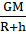Where V = tangential velocity

G = gravitational constant

M = mass of earth

h = height of satellite above earth surface

(b) The initial velocity (during launching) of the Mangalyaan, must be greater than escape velocity of the earth.

Explanation: Spacecrafts should escape the earth’s gravitational force to travel to outer space. Escape velocity is the minimum speed required by an object i.e. satellite to escape from the gravitational force of the earth. The minimum velocity of satellite should be 11.2 km/sec.

Question 2.

State with reasons whether the following sentences are true or false

a. If a spacecraft has to be sent away from the influence of earth’s gravitational field, its velocity must be less than the escape velocity.

b. The escape velocity on the moon is less than that on the earth.

c. A satellite needs a specific velocity to revolve in a specific orbit.

d. If the height of the orbit of a satellite increases, its velocity must also increase.

(a) False

Explanation: Spacecrafts should escape the earth’s gravitational force to travel to outer space. Escape velocity is the minimum speed required by an object i.e. satellite to escape from the gravitational force of the earth. So its speed should be more than the escape velocity.

(b) True

Explanation: The mass of moon is less than the mass of earth so its gravitational pull is less hence escape velocity on moon is less as compared to earth.

(c) True

Explanation: A satellite requires a specific velocity to revolve in a specific orbit called critical velocity which acts as a tangential direction to the orbit.

(d) False

Explanation: If the height of the orbit of a satellite increases, its velocity decreases. As tangential velocity of satellite is inversely proportional to the height of orbit of satellite from earth’s surface.

V= √Where V = tangential velocity

G = gravitational constant

M = mass of earth

h = height of satellite above earth surface

Question 3.

What is meant by an artificial satellite? How are the satellites classified based on their functions?

A manmade object revolves around the earth or any other planet in a fixed orbit is called an artificial satellite.

Based on their function satellites are of various types-

a. Weather satellite- Study and prediction of weather.

b. Communication satellite – Establish communication between different location of the world.

c. Broadcast satellite – Telecasting of tv programmes.

d. Navigational satellite – Fix the location of any place on the earth’s surface.

e. Military satellite – Collect information for security aspects.

f. Earth observation satellite – Study of forests, deserts, oceans, polar ice etc.

Question 4.

What is meant by the orbit of a satellite? On what basis and how are the orbits of artificial satellites classified?

An orbit is a regular, repeating path that satellite takes around the earth to revolve. Depending on the height of the satellite’s orbit above the earth surface, the orbit of satellites are classified as follows-

i. High earth orbits- If the height of the satellite’s orbit above the earth’s surface is greater than or equal to 35780 km, the orbit is called high earth orbit. These satellites almost take 24 hours to complete one revolution. These are also called geosynchronous satellites. They are used in meterology, carry signals for telephone, TV, radio etc.

ii. Medium earth orbits- If height of the satellite orbit above the earth’s surface is in between 2000 km and 35780 km the orbits are called medium earth orbits.

iii. Low earth orbits – If the height of the satellite orbit above the earth’s surface is in between 180 km and 2000 km the orbits are called low earth orbits. They are used for scientific experiments and atmospheric studies.

Question 5.

Why are geostationary satellites not useful for studies of polar regions?

Geostationary satellites orbit is above the equator so they are not useful for studies of polar regions.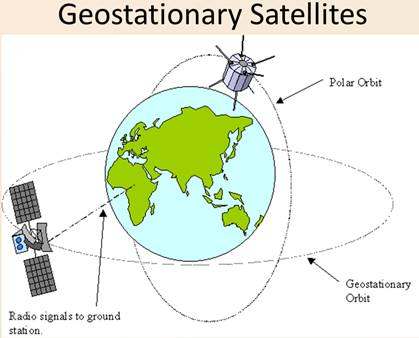Question 6.

What is meant by satellite launch vehicles? Explain a satellite launch vehicle developed by ISRO with the help of a schematic diagram.

Satellite launch vehicles are used to place the satellites in their specific orbits. The function of this vehicle is based on Newton’s third law of motion. ISRO has developed PSLV [ polar satellite launch vehicle ]. It consists of an engine which uses solid fuel that provides initial thrust which provides certain velocity and takes it to certain height. There are four stages which uses solid and liquid fuel alternatively. Once the first stage fuel is used up the engine and first stage gets detached from the main body and falls into sea or unpopulated land. The weight got reduced and it moves with more high speed. Once the fuel in first stage is exhausted the fuel in second stage is ignited and so on.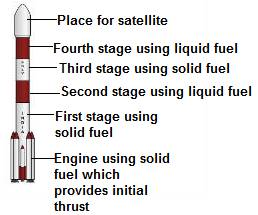Question 7.

Why it is beneficial to use satellite launch vehicles made of more than one stage?

The fuel forms a major portion of the total weight of the launch vehicle so vehicle has to carry a large weight with it. So to overcome these problems launch vehicles are made up of more than one stage so that the fuel in the stage when exhausted it gets detached from the main body step by step and weight is reduced so that it can move with high speed.

Question 8.

Complete the following table.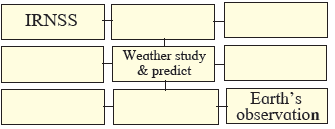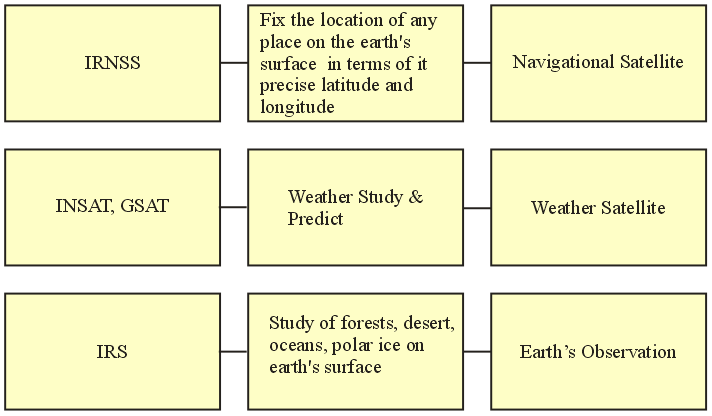IRNSS: Fix the location of any place on the earth’s surface in terms of it precise latitude and longitude : Navigational Satellite.

INSAT, GSAT : Weather study & predict: Weather satellite.

IRS: Study of forests, desert, oceans, polar ice on earth’s surface: Earth’s observation.

Question 9.

Solve the following problems.

If mass of a planet is eight times the mass of the earth and its radius is twice the radius of the earth, what will be the escape velocity for that planet?

Mass of planet M = 8 x mass of earth = 8 x 6 x 1024 kg

Radius of planet R = 2 x radius of earth = 2 x 6.4 x 106 m

G = gravitational constant = 6.67 x 10-11 N m2/kg2

So escape velocity = √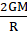Escape velocity =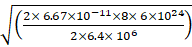= 22.4 km/sec.

So escape velocity of the planet will be 22.4 km/sec

Question 10.

Solve the following problems.

How much time a satellite in an orbit at height 35780 km above earth’s surface would take, if the mass of the earth would have been four times its original mass?

Given G = gravitational constant = 6.67 x 10-11 N m2/kg2

M mass of earth = 4 x 6 x 1024 kg

R = 6400 km

h = height of satellite above earth’s surface = 35780 km

R+h = 6400 + 35780 = 42180 km = 42180 x 103 m

v = ?

we know

v = √=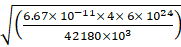v = 6.16 km/s

We know v = distance/time

= circumference/time

= 2πr/T

T =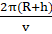T =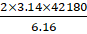T = 43001.68 sec

T = 11.94 hr or approximately 12 hours.

Question 11.

Solve the following problems.

If the height of a satellite completing one revolution around the earth in T seconds is h1 meter, then what would be the height of a satellite taking 2√2 T seconds for one revolution?

Given:
The height of the satellite completing one revolution around the earth in T seconds.

let the height be x of a satellite taking 2√2 T seconds for one revolution.

From Kelper’s third law of motion:

The square of the time period of revolution of the planet around the sun is directly proportional to the cube semi-major axis of its elliptical orbit.

T ∝ r3/2

Where
T is the time period
r is the radius of the satellite

Thus we can say,
T = K r3/2 ....(i)

Here, r1 = r when, T1 = t

We get,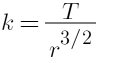Then, if T2 = 2√2t

Putting the value in the equation(i), we get
2T = K r13/2
Putting the value of K in the above equation, we getOn solving the above equation, we get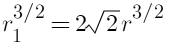Squaring both side and then taking cube-root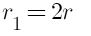.....(ii)

Now r1= R + x(radius of earth + height above earth) and r=R+ h1
so using these relations we get
x=R + 2h1